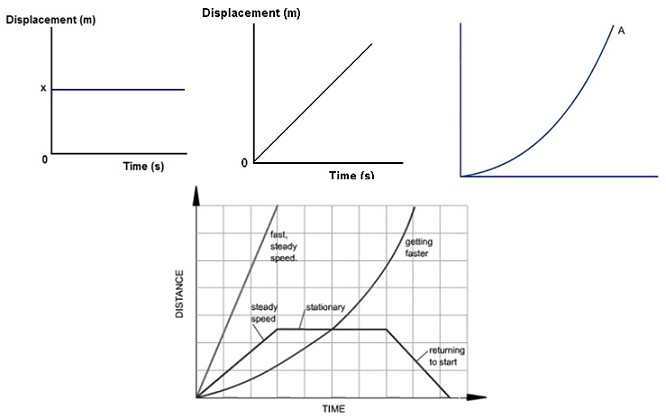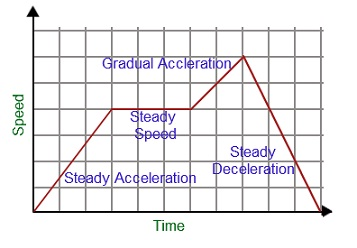#### Motion in a Straight Line, Physics tutorial

Introduction:

The word motion is generally related by the movement of objects whenever forces act on them. You can utilize the word to explain any object which is not at rest such as an airplane in flight or a man walking all along the road.

Motion can occur in one, two or three dimensions. Whenever a body moves all along a straight line, we state of one dimensional motion. Two dimensional motions is a motion in a plane. For illustration: the motion of footballers on a field. Three dimensional motions occur in space. Illustration: The flight of mosquito in a room.

Types of Motion:

There are basically four main kinds of motion:

1) Random motion:

An object is stated to undergo random motion if it moves in an irregular way without favored direction or orientation.

Illustrations:

• Movement of the players in a football field.
• Brownian motion, that is, an irregular motion of particles hanged in water or of smoke particles hanged in air.

2) Linear/Translational Motion:

Whenever rigid objects move away from one point in space to the other devoid of rotating, the motion is stated to be translational. In translational motion, you will observe that each and every point in the body remains comparatively fixed to one other. Each and every part of an object undergoing pure translational motion follows the similar path.

Illustrations:

• An insect crawling from one point to the other.
• Whenever you move from your home to the school.
• A car travelling from Enugu to Abuja, all along the road.

3) Rotational Motion:

An object experiences rotational motion whenever its movement needs its complete turning. It signifies that all the points in the body move in concentric circles like the point in a rotating wheel. The centre of such circles all lie on the line termed as the axis of rotation.

Illustrations:

• The earth spinning around its axis.
• Rotational motion of a record disc.
• The movement of blades of a fan.

4) Oscillatory (or Vibrational) Motion:

An object experience oscillatory motion whenever its movement is periodic. That is, the object experiences a 'to and fro' movement around an axis, reversing the direction of its motion and returning regularly to its original place.

Illustration:

• The motion of a pendulum as it swings backward and forward.

Relative Motion:

Relative motion is the computation of the motion of an object with regard to some other moving object. Therefore, the motion is not computed through the reference to earth; however is the velocity of the object in reference to the other moving object as when it were in a static state.

For illustration: A ball thrown upward while in a moving object like a bus, would be traveling similar speed with respect to the bus and would fall again in relation to that speed. When the ball stopped in mid-air rather, the ball would hit the back of the bus prior to falling to the floor. In this illustration, the relative motion of the ball was illustrated in respect to the bus. When the driver turned left, the ball would as well be moving left even when it was stationary on the floor. When the ball was illustrated in relation to a distinct point, like a pedestrian on the side-walk and the bus was moving at 40 mph, it would be traveling faster than the pedestrian.

Parameters employed in explaining Motion:

The parameters utilized in explaining motion are time, displacement (that is, distance in a particular direction), speed, velocity and acceleration.

Concept of Speed:

In explaining the motion of a body, we take both the distance and time it takes to cover that distance. The rate at which a body covers a distance is termed as the speed of the body.

Speed = Distance/Time

Symbolically, speed (v) = distance (s)/time (t)

v = s/t

Concept of Velocity:

Velocity is the rate of change of displacement by means of time or the rate of change of distance with time or the rate of change of distance with time in a particular direction.

Velocity = displacement/time

v = s/t

Concept of Acceleration/Retardation:

An object might be moving by non-uniform velocity (that is, changing velocity). The change could be increase or decrease in velocity. Whenever the velocity of a moving object is rising, the object is stated to be accelerating. Whenever it is reducing, the object is stated to be retarding. Acceleration (or retardation) is stated as the rate of change of velocity with time.

Particularly,

Acceleration (a) = increase in velocity (v)/time taken (t)

Acceleration (a) = velocity change/ time taken to make the change

a = v/t

Retardation (- a) = velocity change/time taken to make the change

- a = v/t

S.I. Unit = metre per second squared (ms-2)

Distance/Displacement-Time graph:

The displacement/distance-time graph of an object can be drawn by taking the time taken all along the x-axis and the distance travelled all along the y-axis. The ratio of the distance travelled and the time-taken will give the speed of the object. Thus, the slope of the distance-time graph provides the speed of the particular object. That is, by employing this graph one can determine the speed of the object.

The displacement/distance-time graph under dissimilar conditions is stated below:

a) If object is at rest:

At rest, the position of object will be constant, that is, the object will not change its position with respect to the time. It remains at similar distance at all time. The distance-time graph for the object at rest is a straight line parallel to the time axis. The slope of graph will be (0) zero.

b) If object moving with a uniform speed:

If the object covers equivalent distance in equal time interval, then it is stated to encompass a uniform speed. The distance-time graph for object moving by uniform speed is a straight line containing all the points falls on it that will make an angle with the x-axis. The slope of graph will provide the speed of an object and the slope should be similar everywhere in the straight line.

c) If object moving with non-uniform speed:

If a body moves with non-uniform speed it covers unequal distance in equivalent time intervals time. The distance-time graph for a body that is moving by non-uniform speed is not a straight line. This is a curve. The speed of object will change with respect to time.Speed/Velocity-Time graph:

The geometrical relationship among the speed of an object and the time-taken by the object is termed as the speed-time graph. The speed-time graph of an object can be drawn by taking the time taken all along the x-axis and the speed all along the y-axis. The ratio of speed and the time taken will provide the acceleration of the object. Thus, the slope of the speed-time graph provides the acceleration of the particular object. That is, by employing this graph one can determine the acceleration of an object.

• The steepness on the graph represents that the object is having more acceleration.
• When the graph is horizontal, it signifies that the object is moving with constant speed and the acceleration is zero.
• Whenever graph is coming down, it represents or exhibits that the moving object is slowing down.Tutorsglobe: A way to secure high grade in your curriculum (Online Tutoring)

Expand your confidence, grow study skills and improve your grades.

Since 2009, Tutorsglobe has proactively helped millions of students to get better grades in school, college or university and score well in competitive tests with live, one-on-one online tutoring.

Using an advanced developed tutoring system providing little or no wait time, the students are connected on-demand with a tutor at www.tutorsglobe.com. Students work one-on-one, in real-time with a tutor, communicating and studying using a virtual whiteboard technology.  Scientific and mathematical notation, symbols, geometric figures, graphing and freehand drawing can be rendered quickly and easily in the advanced whiteboard.

Free to know our price and packages for online physics tutoring. Chat with us or submit request at info@tutorsglobe.com